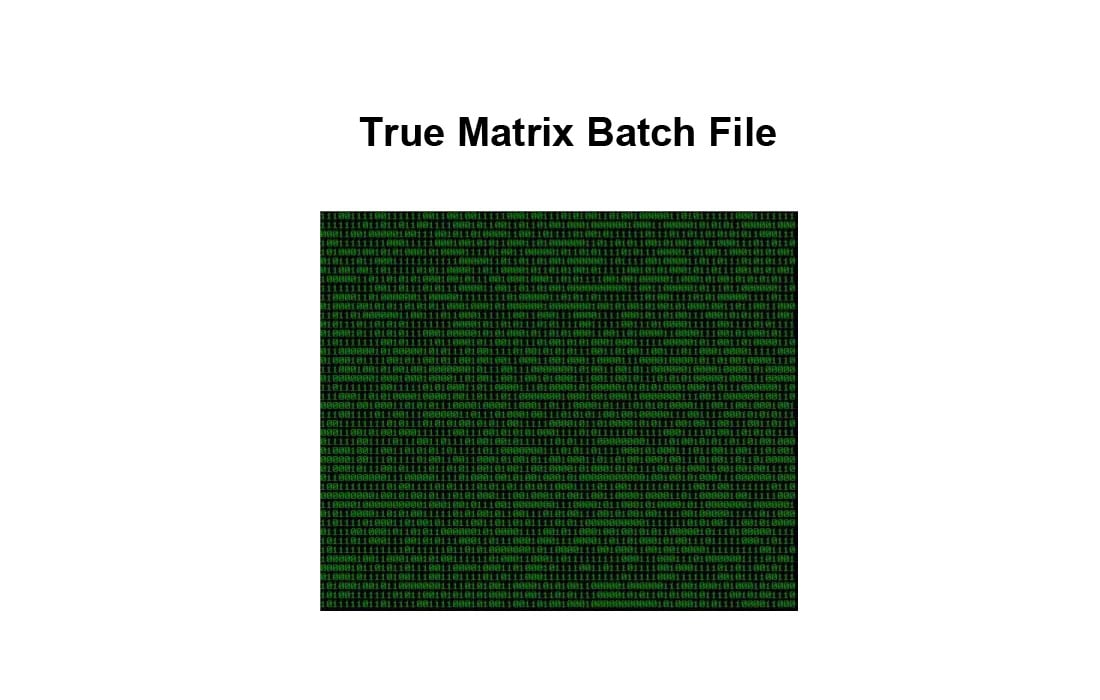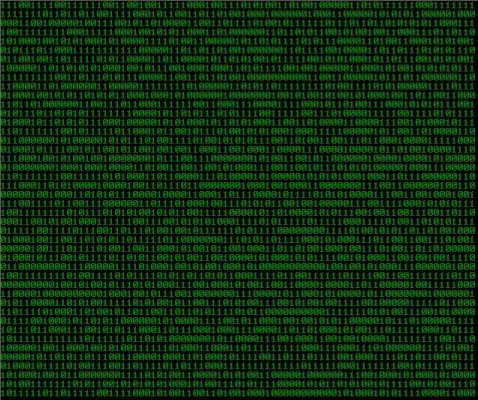# True Matrix Batch FileFor a long time my bud and i had been working on a matrix script. We had been asked buy someone in our computer tech class to come up with a matrix batch, and for the past 3 months or so, we’ve been working on it. We found out how to generate a random 1 or 0, but we couldn’t figure out how to make it truly random per digit, only per line. Earlier today we figured it out. Alot fo matrix batch file online claim to be a true matrix batch. And honestly they are pretty cool. But this batch file is a TRUE random generation of 1’s and 0’s, without any skipping or gaps like most of the others i found have.

Without further adue, i present, the matrix batch.``` @echo off color 2 :SHOUT set /a a=%random%*2/32767 set /a b=%random%*2/32767 set /a c=%random%*2/32767 set /a d=%random%*2/32767 set /a e=%random%*2/32767 set /a f=%random%*2/32767 set /a g=%random%*2/32767 set /a h=%random%*2/32767 set /a i=%random%*2/32767 set /a j=%random%*2/32767 set /a k=%random%*2/32767 set /a l=%random%*2/32767 set /a m=%random%*2/32767 set /a n=%random%*2/32767 set /a o=%random%*2/32767 set /a p=%random%*2/32767 set /a q=%random%*2/32767 set /a r=%random%*2/32767 set /a s=%random%*2/32767 set /a t=%random%*2/32767 set /a u=%random%*2/32767 set /a v=%random%*2/32767 set /a w=%random%*2/32767 set /a x=%random%*2/32767 set /a y=%random%*2/32767 set /a z=%random%*2/32767 echo %a%%d%%c%%b%%b%%f%%a%%e%%f%%g%%h%%c%%z%%x%%y%%f%%e%%r%%b%%m%%z%%p%%k%%u%%i%%g%%s%%a%%d%%l%%o%%p%%w%%t%%y%%b%%n%%f%%u%%s%%p%%s%%t%%u%%g%%h%%s%%l%%r%%s%%q%%w%%e%%r%%t%%v%%b%%n%%o%%u%%i%%l%%p%%s%%f%%e%%c%%x%%z%%b%%h%%r%%u%%l%%o%%m%%e%%e%%s% goto SHOUT ```